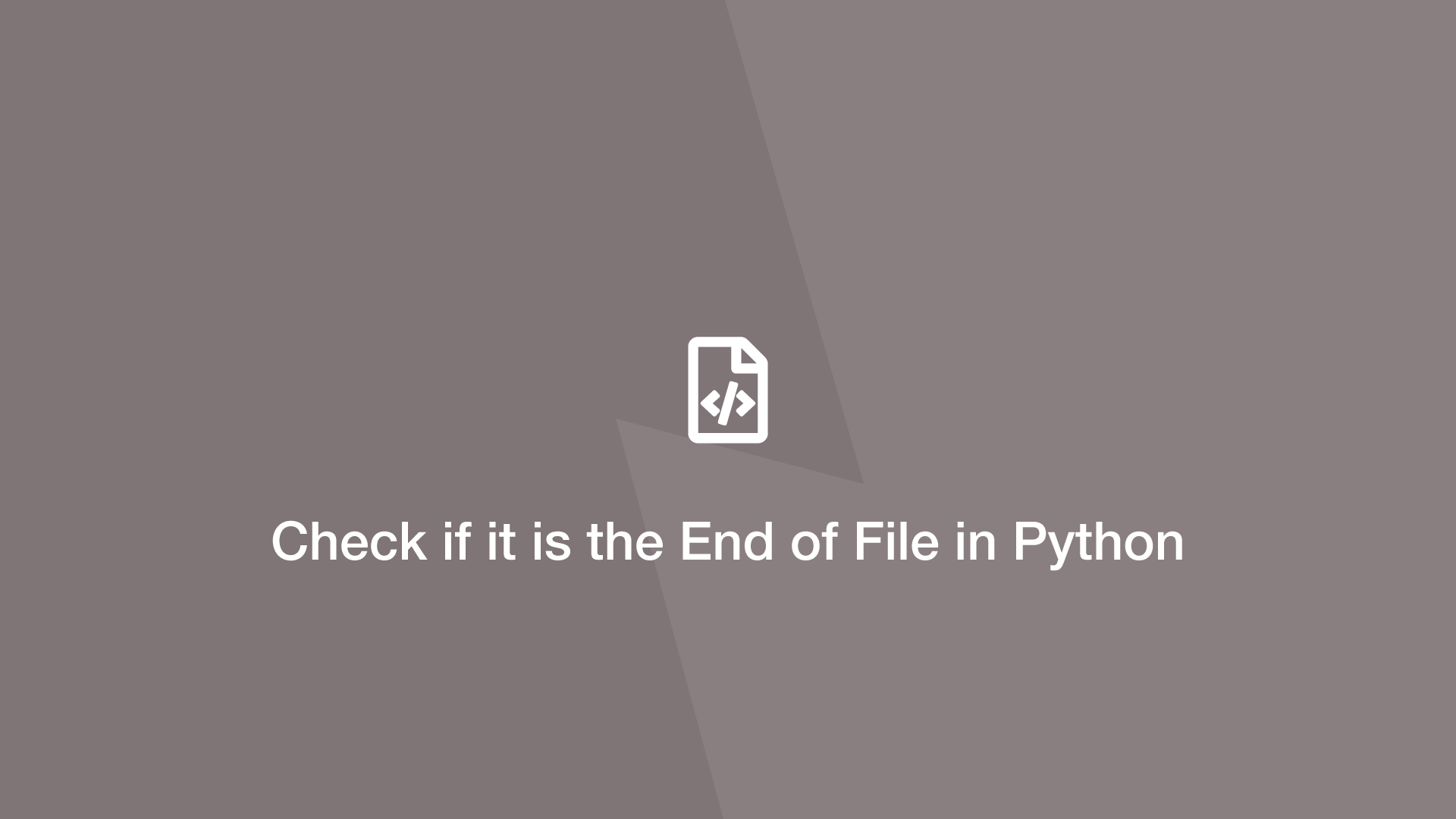# How to Check if it is the End of File in PythonIf the end of the file (EOF) is reached in Python the data returned from a read attempt will be an empty string.

Let's try out two different ways of checking whether it is the end of the file in Python.

## Calling the Read Method Again

We can call the Python `.read()` method again on the file and if the result is an empty string the read operation is at EOF.

file.txt
``````Some content.
Another line of content.
``````
``````open_file = open("file.txt", "r")

if eof == '':
print('EOF')
``````
``````EOF
``````

## Read Each Line in a while Loop

Another option is to read each line of the file by calling the Python `readline()` function in a `while` loop. When an empty string is returned we will know it is the end of the file and we can perform some operation before ending the while loop.

file.txt
``````Some content.
Another line of content.
``````
``````path = 'file.txt'

file = open(path, 'r')

x = True

while x:
``````EOF